#### Rotation

The robot,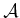, can be rotated counterclockwise by some angle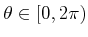by mapping every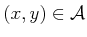as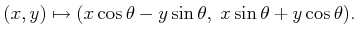(3.30)

Using a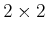rotation matrix,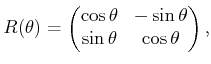(3.31)

the transformation can be written as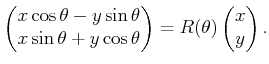(3.32)

Using the notation of Section 3.2.1,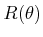becomes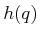, for which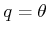. For linear transformations, such as the one defined by (3.32), recall that the column vectors represent the basis vectors of the new coordinate frame. The column vectors ofare unit vectors, and their inner product (or dot product) is zero, indicating that they are orthogonal. Suppose that theand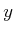coordinate axes, which represent the body frame, are painted'' on. The columns ofcan be derived by considering the resulting directions of the- and-axes, respectively, after performing a counterclockwise rotation by the angle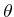. This interpretation generalizes nicely for higher dimensional rotation matrices.

Note that the rotation is performed about the origin. Thus, when defining the model of, the origin should be placed at the intended axis of rotation. Using the semi-algebraic model, the entire robot model can be rotated by transforming each primitive, yielding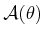. The inverse rotation,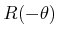, must be applied to each primitive.

Steven M LaValle 2020-08-14